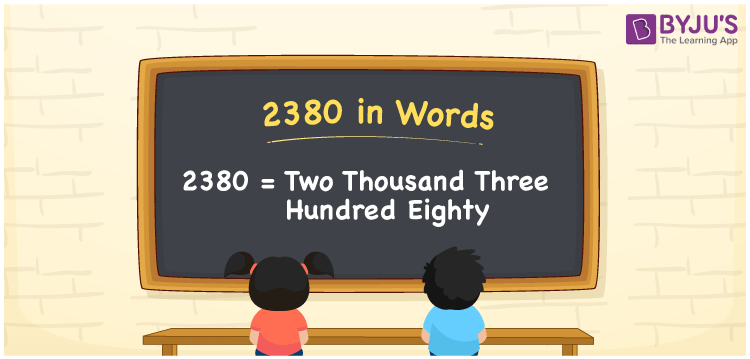# 2380 in Words

2380 in words is written as Two thousand three hundred eighty. In both the International System of Numerals and the Indian System of Numerals, 2380 in words, is written as Two thousand three hundred eighty. The number 2380 is a Cardinal Number as it represents some quantity. For example, “that item costs 2380 rupees”.

 2380 in Words Two thousand three hundred eighty Two thousand three hundred eighty in Number 2380

## 2380 in English Words

2380 in English words is read as “Two thousand three hundred eighty”.## How to Write 2380 in Words?

To write 2380 in words, we shall use the place value chart. In the place value chart, put 2 in the thousands, 3 in the hundreds, 8 in the tens, and 0 in the ones, respectively. Let us make a place value chart to write the number 2380 in words.

 Thousands Hundreds Tens Ones 2 3 8 0

Thus, we can write the expanded form as

2 × Thousand + 3 × Hundred + 8 × Ten + 0 × One

= 2 × 1000 + 3 × 100 + 8 × 10 + 0 × 1

= 2000 + 300 + 80 + 0

= 2380

= Two thousand three hundred eighty.

2380 is a natural number which is the successor of 2379 and the predecessor of 2381.

2380 in words – Two thousand three hundred eighty

• Is 2380 an odd number? – No
• Is 2380 an even number? – Yes
• Is 2380 a perfect square number? – No
• Is 2380 a perfect cube number? – No
• Is 2380 a prime number? – No
• Is 2380 a composite number? – Yes

## Frequently Asked Questions on 2830 in Words

Q1

### How to write 2380 in words?

2380 in words is written as Two thousand three hundred eighty.
Q2

### How to write 2380 in words in the International and Indian System of Numerals?

In both, the system of numerals, 2380 in words, is written as Two thousand three hundred eighty.
Q3

### How to write 2380 in a place value chart?

In the place value chart, write 2 in the thousands, 3 in the hundreds, 8 in the tens, and 0 in the ones, respectively.